# python opencv -详解hough变换检测直线与圆

Hough变换的原理是将特定图形上的点变换到一组参数空间上，根据参数空间点的累计结果找到一个极大值对应的解，那么这个解就对应着要寻找的几何形状的参数（比如说直线，那么就会得到直线的斜率k与常熟b，圆就会得到圆心与半径等等）。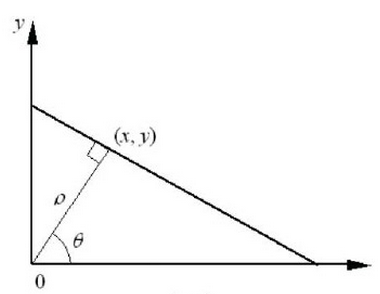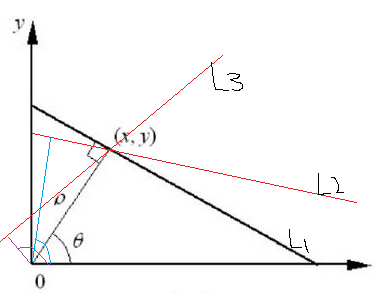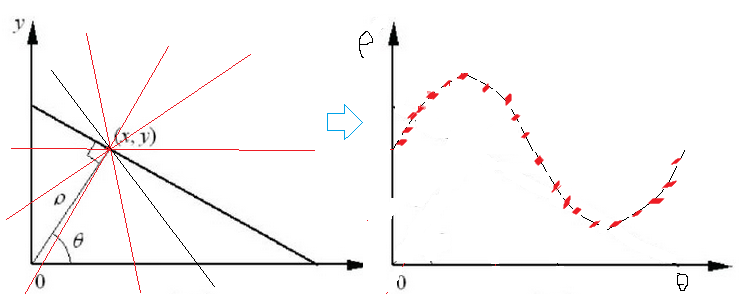ok前面说的是单单这一个点对应的极坐标系下的参数对，那么如果每个点都这么找一圈呢？也就是每个点在参数空间上都对应一系列参数对吧，现在把它们华仔同一个坐标系下会怎么样呢？为了方便，假设在这个直线上取3个点画一下：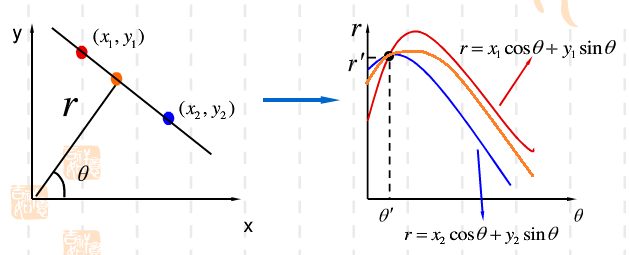（1）将参数空间 (ρ,θ) 量化，赋初值一个二维矩阵M， M(ρ,θ) 就是一个累加器了。
（2）然后对图像边界上的每一个点进行变换，变换到属于哪一组 (ρ,θ) ，就把该组 (ρ,θ) 对应的累加器数加1，这里的需要变换的点就是上面说的经过边缘提取以后的图像了。
（3）当所有点处理完成后，就来分析得到的 M(ρ,θ) ，设置一个阈值T，认为当 M(ρ,θ)>T ，就认为存在一条有意义的直线存在。而对应的 M(ρ,θ) 就是这组直线的参数，至于T是多少，自己去式，试的比较合适为止。
（4）有了 M(ρ,θ) 和点（x,y）计算出来这个直线就ok了。

Opencv中检测直线的函数有cv2.HoughLines()，cv2.HoughLinesP()

import cv2
import numpy as np
import matplotlib.pyplot as plt

gray = cv2.cvtColor(img,cv2.COLOR_BGR2GRAY)#灰度图像
#open to see how to use: cv2.Canny
#http://blog.csdn.net/on2way/article/details/46851451
edges = cv2.Canny(gray,50,200)
plt.subplot(121),plt.imshow(edges,‘gray‘)
plt.xticks([]),plt.yticks([])
#hough transform
lines = cv2.HoughLines(edges,1,np.pi/180,160)
lines1 = lines[:,0,:]#提取为为二维
for rho,theta in lines1[:]:
a = np.cos(theta)
b = np.sin(theta)
x0 = a*rho
y0 = b*rho
x1 = int(x0 + 1000*(-b))
y1 = int(y0 + 1000*(a))
x2 = int(x0 - 1000*(-b))
y2 = int(y0 - 1000*(a))
cv2.line(img,(x1,y1),(x2,y2),(255,0,0),1)

plt.subplot(122),plt.imshow(img,)
plt.xticks([]),plt.yticks([])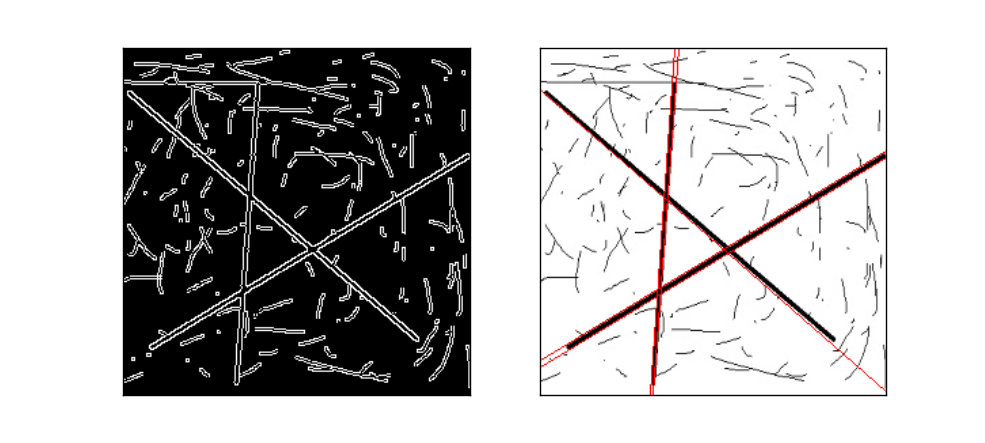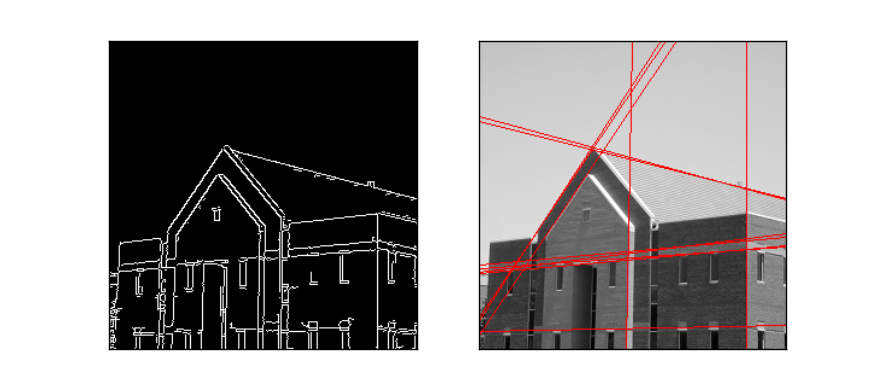import cv2
import numpy as np
import matplotlib.pyplot as plt

gray = cv2.cvtColor(img,cv2.COLOR_BGR2GRAY)#灰度图像
#open to see how to use: cv2.Canny
#http://blog.csdn.net/on2way/article/details/46851451
edges = cv2.Canny(gray,50,200)
plt.subplot(121),plt.imshow(edges,‘gray‘)
plt.xticks([]),plt.yticks([])
#hough transform
lines = cv2.HoughLinesP(edges,1,np.pi/180,30,minLineLength=60,maxLineGap=10)
lines1 = lines[:,0,:]#提取为二维
for x1,y1,x2,y2 in lines1[:]:
cv2.line(img,(x1,y1),(x2,y2),(255,0,0),1)

plt.subplot(122),plt.imshow(img,)
plt.xticks([]),plt.yticks([])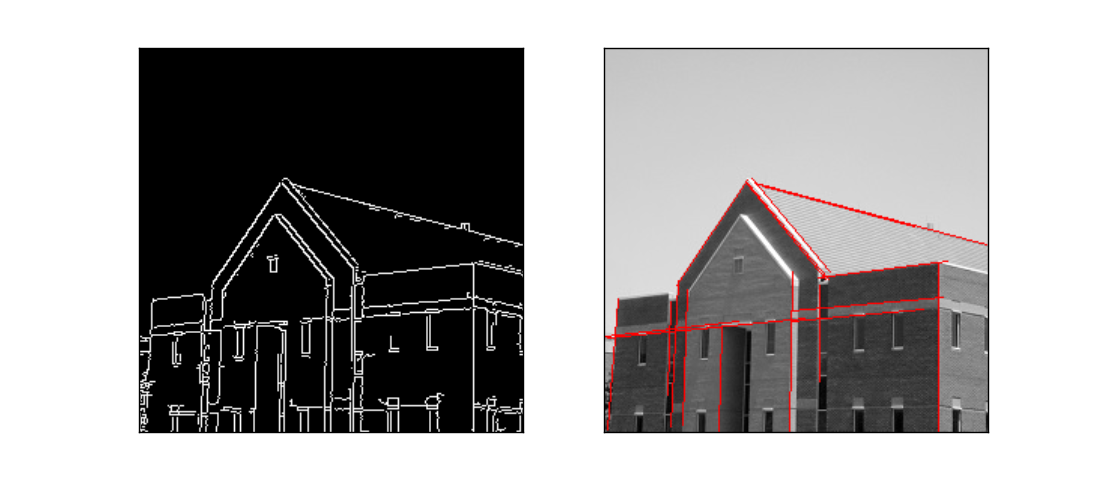Ok说完了直线的检测，再来说说圆环的检测，我们知道圆的数学表示为：

(x?xcenter)2+(y?ycenter)2=r2

import cv2
import numpy as np
import matplotlib.pyplot as plt

gray = cv2.cvtColor(img,cv2.COLOR_BGR2GRAY)#灰度图像

plt.subplot(121),plt.imshow(gray,‘gray‘)
plt.xticks([]),plt.yticks([])
#hough transform
circles = circles1[0,:,:]#提取为二维
circles = np.uint16(np.around(circles))#四舍五入，取整
for i in circles[:]:
cv2.circle(img,(i,i),i,(255,0,0),5)#画圆
cv2.circle(img,(i,i),2,(255,0,255),10)#画圆心

plt.subplot(122),plt.imshow(img)
plt.xticks([]),plt.yticks([])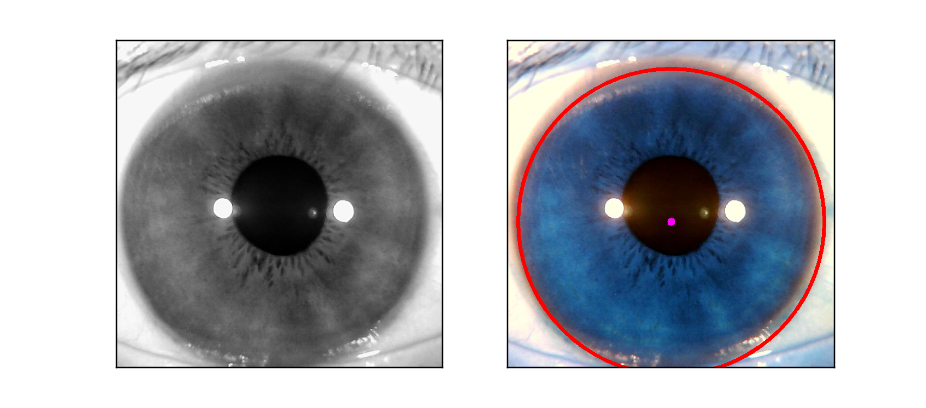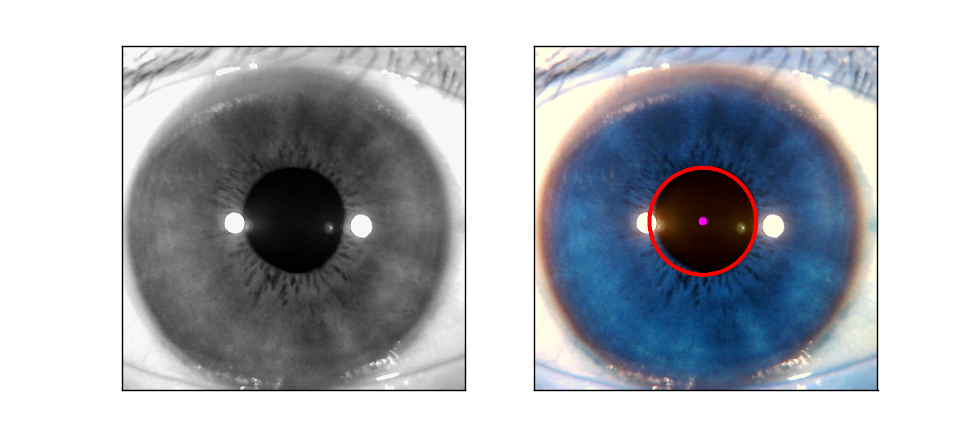Python下opencv使用笔记（十一）（详解hough变换检测直线与圆）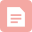博客
Pythonopencv使用笔记（十一）（详解hough变换检测直线
07-236万+博客

05-253943博客
OpenCV-Python 霍夫直线检测-HoughLinesP函数参数
01-064417博客
python OpenCV基础入门教程
07-032513博客

01-083442博客
[图解]cv2.HoughLines() 和 cv2.HoughLinesP()原理和代码

11-276328博客
[OpenCV] HoughLines和HoughLinesP的区别不同效果展示
06-171万+博客
OpenCV自学笔记27. Hough变换检测直线
09-037049博客
Hough变换 理解 & 直线检测检测代码
11-24367博客
python 直线检测_利用OpencvHoughline方法实现直线检测
12-05316博客

11-303万+博客
hough变换直线检测_【从零学习OpenCV 4】霍夫变换原理及直线检测
12-01516博客
【图像处理】——Python霍夫变换直线检测（主要是两个函数Houghlines&HoughlinesP）
09-127925博客
python压线检测_Python cv2 HoughLines网格线检测
12-10239博客
Pythonopencv hough变换
03-31362博客
python opencv入门 Hough变换（28）
08-231万+博客
python实现opencv学习十八：hough变换检测
06-29133博客
Python+opencv — cv2.HoughCircles（） 检测，并顺时针给编序
08-251799博客
PythonOpenCV中的霍夫变换——cv2.HoughCircles()
07-264016博客
cv2做hough直线检测
07-172100

### “相关推荐”对你有帮助么？

•非常没帮助
•没帮助
•一般
•有帮助
•非常有帮助点击重新获取扫码支付余额充值